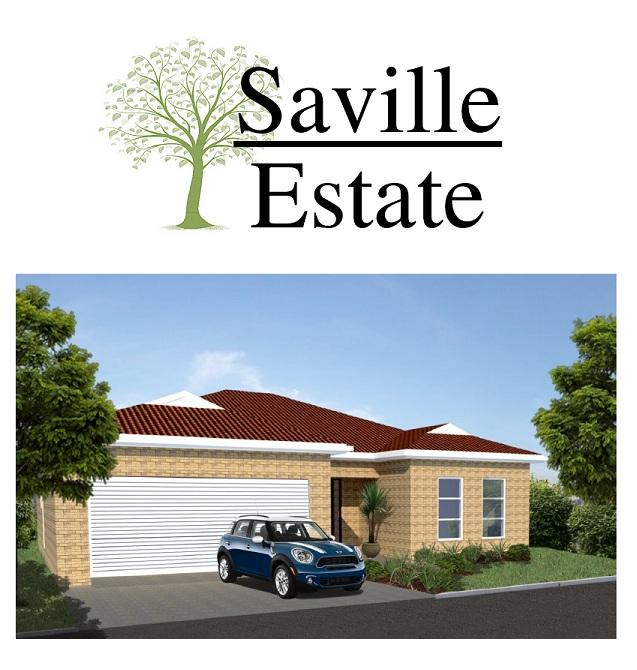Share

ref # 464#### R 1 290 000 (75 438 EUR*)

select a 2nd currency

3
2
2

##### Sonkring (Saville Estate) – New security estate in Sonkring (Brackenfell) from R1 290 000

Sonkring – Saville Estate (“Turn Key” Development)

Unit 1 = (Plan A) @ R1 560 000 (Plot = 621m²)
Unit 2 = (Plan A) @ R1 560 000 (Plot = 360m²)
Unit 3 = (Plan A) @ R1 560 000 (Plot = 360m²)
Unit 4 = (Plan C) @ R1 380 000 (Plot = 385m²)
Unit 5 = (Plan A) @ R1 545 000 (Plot = 541m²)
Unit 6 = (Plan A) @ R1 490 000 (Plot = 330m²)
Unit 7 = (Plan A) @ R1 490 000 (Plot = 330m²)
Unit 8 = (Plan A) @ R1 555 000 (Plot = 511m²)
Unit 9 = (Plan A) @ R1 565 000 (Plot = 392m²)
Unit 10 = (Plan A) @ R1 565 000 (Plot = 391m²)
Unit 11 = (Plan A) @ R1 560 000 (Plot = 551m²)
Unit 12 = (Plan A) @ R1 590 000 (Plot = 468m²)
Unit 13 = (Plan A) @ R1 495 000 (Plot = 300m²)
Unit 14 = (Plan A) @ R1 490 000 (Plot = 300m²)
Unit 15 = (Plan B) @ R1 290 000 (Plot = 295m²)

Costs include :

Plot
Water and electricity connections (pre-paid electricity meter)
NHBRC fees

Don’t miss out…

## Message Agent

+27 (0)82 774 4562
leana@leanameyerproperties.... leana@leanameyerproperties.co.za